# Tonelli plane variation

(diff) ← Older revision | Latest revision (diff) | Newer revision → (diff)

A numerical characteristic of a function of two variables, by means of which one defines the class of functions of bounded variation in the sense of Tonelli. Suppose thatis a function given on the rectangle. Assume that the functions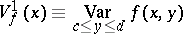and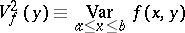are Lebesgue measurable (the first on the interval, the second on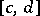). If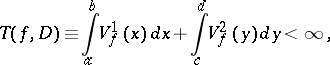then one says that the functionhas bounded (finite, or: is of bounded (finite)) Tonelli plane variation on the rectangle, and the class of all such functions is denoted by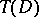. This definition was proposed by L. Tonelli (cf. , ). For continuous functions, however, another characterization (in terms of the Banach indicatrix) of the classcan be found in an earlier paper of S. Banach . If the functionis continuous on the rectangle, then the surfacehas finite area if and only ifbelongs to(cf. Tonelli theorem).

How to Cite This Entry:
Tonelli plane variation. Encyclopedia of Mathematics. URL: http://encyclopediaofmath.org/index.php?title=Tonelli_plane_variation&oldid=16914
This article was adapted from an original article by B.I. Golubov (originator), which appeared in Encyclopedia of Mathematics - ISBN 1402006098. See original article